# 1 Prehistoric goblets of Thailand

We will use PCA to understand how the dimensions of goblets relate to each other. The data consist of six height and width measurements in cm on each of 25 prehistoric goblets found in Thailand: MouthW, TotalW, TotalH, BaseW, StemW, and StemH. The image below gives an example of a goblet, possibly not from prehistoric Thailand.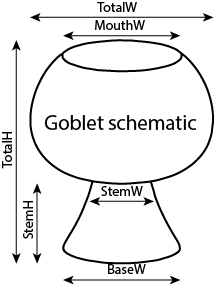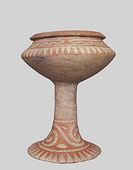library(tidyverse)

dat_goblets <-
# rename columns from x1-x6 to meaningful names
, skip = 1
, col_names = c("MouthW", "TotalW", "TotalH", "BaseW", "StemW", "StemH")
)
head(dat_goblets, 3)
# A tibble: 3 x 6
MouthW TotalW TotalH BaseW StemW StemH
<dbl>  <dbl>  <dbl> <dbl> <dbl> <dbl>
1     13     21     24    14     7     8
2     14     14     24    19     5     9
3     19     23     24    20     6    12

## 1.1(2 p) PCA on original scale, PC1

A PCA of the goblets shows that the major source of variation among the goblets is due to differences in size, as measured by a weighted linear combination of the six features.

goblets_pca <-
princomp(
~ MouthW + TotalW + TotalH + BaseW + StemW + StemH
, data = dat_goblets
, cor = TRUE
)
summary(goblets_pca)
Importance of components:
Comp.1    Comp.2     Comp.3     Comp.4     Comp.5      Comp.6
Standard deviation     2.0663218 1.0445535 0.62242573 0.37725654 0.25547576 0.210280768
Proportion of Variance 0.7116143 0.1818487 0.06456896 0.02372042 0.01087798 0.007369667
Cumulative Proportion  0.7116143 0.8934630 0.95803194 0.98175236 0.99263033 1.000000000
print(loadings(goblets_pca), cutoff = 0) # to show all values

Comp.1 Comp.2 Comp.3 Comp.4 Comp.5 Comp.6
MouthW  0.366  0.487  0.612  0.336  0.278  0.252
TotalW  0.452 -0.036 -0.373  0.663 -0.099 -0.453
TotalH  0.411 -0.442 -0.322 -0.005  0.386  0.619
BaseW   0.462 -0.114  0.164 -0.545  0.386 -0.548
StemW   0.297  0.682 -0.492 -0.360 -0.217  0.167
StemH   0.438 -0.297  0.336 -0.141 -0.753  0.141

Comp.1 Comp.2 Comp.3 Comp.4 Comp.5 Comp.6
Proportion Var  0.167  0.167  0.167  0.167  0.167  0.167
Cumulative Var  0.167  0.333  0.500  0.667  0.833  1.000

As a starting point, briefly describe the evidence below that indicates that PC1 is interpretted as the variability in overall size of the goblets.

### 1.1.1 Solution

If I were primarily interested in the variation in shapes across goblets (instead of sizes), a sensible strategy might be to transform the data by dividing each measurement by the sum of the measurements on a goblet (there are other strategies). The code below creates six size-adjusted (standardized proportions) variables.

# create MouthWs-StemHs within the gobets data.frame
dat_goblets <-
dat_goblets %>%
mutate(
MouthWs = MouthW / (MouthW + TotalW + TotalH + BaseW + StemW + StemH)
, TotalWs = TotalW / (MouthW + TotalW + TotalH + BaseW + StemW + StemH)
, TotalHs = TotalH / (MouthW + TotalW + TotalH + BaseW + StemW + StemH)
, BaseWs  = BaseW  / (MouthW + TotalW + TotalH + BaseW + StemW + StemH)
, StemWs  = StemW  / (MouthW + TotalW + TotalH + BaseW + StemW + StemH)
, StemHs  = StemH  / (MouthW + TotalW + TotalH + BaseW + StemW + StemH)
)
str(dat_goblets)
Classes 'spec_tbl_df', 'tbl_df', 'tbl' and 'data.frame':    25 obs. of  12 variables:
$MouthW : num 13 14 19 17 19 12 12 12 11 11 ...$ TotalW : num  21 14 23 18 20 20 19 22 15 13 ...
$TotalH : num 24 24 24 16 16 24 22 25 17 14 ...$ BaseW  : num  14 19 20 16 16 17 16 15 11 11 ...
$StemW : num 7 5 6 11 10 6 6 7 6 7 ...$ StemH  : num  8 9 12 8 7 9 10 7 5 4 ...
$MouthWs: num 0.149 0.165 0.183 0.198 0.216 ...$ TotalWs: num  0.241 0.165 0.221 0.209 0.227 ...
$TotalHs: num 0.276 0.282 0.231 0.186 0.182 ...$ BaseWs : num  0.161 0.224 0.192 0.186 0.182 ...
$StemWs : num 0.0805 0.0588 0.0577 0.1279 0.1136 ...$ StemHs : num  0.092 0.1059 0.1154 0.093 0.0795 ...
# Correlation matrix
#dat_goblets %>% select(MouthWs:StemHs) %>% cor() %>% print(digits = 3)

# Scatterplot matrix
library(ggplot2)
library(GGally)
p <- ggpairs(dat_goblets %>% select(MouthWs:StemHs))
print(p)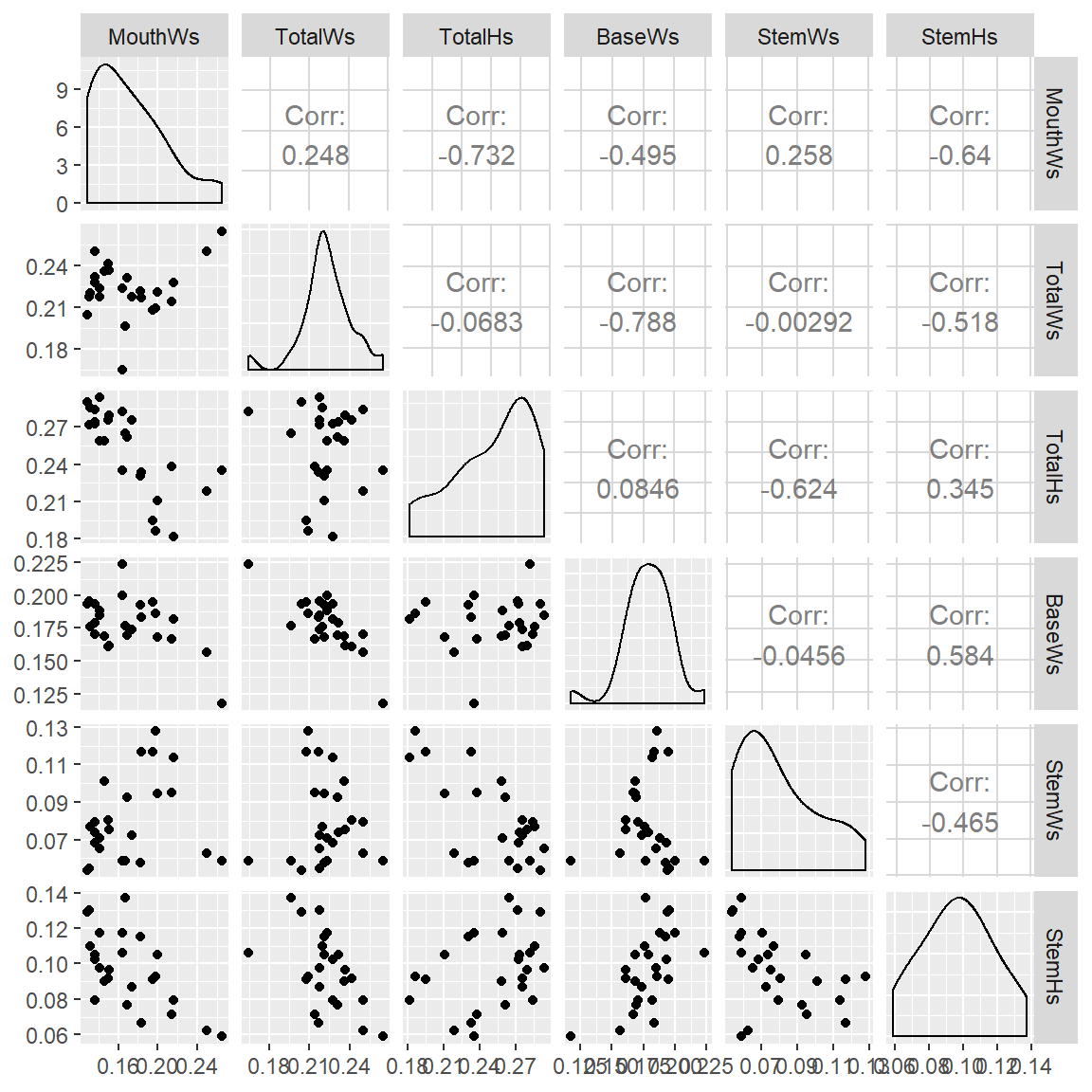In the scatterplot matrix of the size-adjusted measurements describe qualitatively what you see.

## 1.3(3 p) PC interpretations

Do a PCA on the standardized size-adjusted measurements (using the correlation matrix for the size-adjusted measurements).

goblets_pca_s <-
princomp(
~ MouthWs + TotalWs + TotalHs + BaseWs + StemWs + StemHs
, data = dat_goblets
, cor = TRUE
)
summary(goblets_pca_s)
Importance of components:
Comp.1    Comp.2    Comp.3     Comp.4    Comp.5 Comp.6
Standard deviation     1.741076 1.2790076 0.8370514 0.64427244 0.4658901      0
Proportion of Variance 0.505224 0.2726434 0.1167758 0.06918116 0.0361756      0
Cumulative Proportion  0.505224 0.7778674 0.8946432 0.96382440 1.0000000      1
print(loadings(goblets_pca_s), cutoff = 0) # to show all values

Comp.1 Comp.2 Comp.3 Comp.4 Comp.5 Comp.6
MouthWs  0.474  0.156  0.607  0.219  0.075  0.574
TotalWs  0.358 -0.513 -0.192 -0.506 -0.474  0.302
TotalHs -0.375 -0.506 -0.262  0.489  0.176  0.515
BaseWs  -0.418  0.466 -0.022  0.088 -0.713  0.303
StemWs   0.297  0.485 -0.680 -0.099  0.297  0.340
StemHs  -0.493  0.059  0.252 -0.663  0.378  0.328

Comp.1 Comp.2 Comp.3 Comp.4 Comp.5 Comp.6
Proportion Var  0.167  0.167  0.167  0.167  0.167  0.167
Cumulative Var  0.167  0.333  0.500  0.667  0.833  1.000

Interpret all the principal components (treating relatively small loadings as zeroes) by completing the sentences below by replacing “[answer here]”.

### 1.3.1 Solution

PC1 explains 50.5% of the total variation, a contrast between [answer here].

$\text{PC}1 = 0.47 \text{ MouthWs} +0.36 \text{ TotalWs} +-0.38 \text{ TotalHs} +-0.42 \text{ BaseWs} +0.3 \text{ StemWs} +-0.49 \text{ StemHs}$

PC2 explains 27.3% of the total variation, a contrast between [answer here].

$\text{PC}2 = 0.16 \text{ MouthWs} +-0.51 \text{ TotalWs} +-0.51 \text{ TotalHs} +0.47 \text{ BaseWs} +0.49 \text{ StemWs} +0.059 \text{ StemHs}$

PC3 explains 11.7% of the total variation, a contrast between [answer here].

$\text{PC}3 = 0.61 \text{ MouthWs} +-0.19 \text{ TotalWs} +-0.26 \text{ TotalHs} +-0.022 \text{ BaseWs} +-0.68 \text{ StemWs} +0.25 \text{ StemHs}$

PC4 explains 6.92% of the total variation, a contrast between [answer here].

$\text{PC}4 = 0.22 \text{ MouthWs} +-0.51 \text{ TotalWs} +0.49 \text{ TotalHs} +0.088 \text{ BaseWs} +-0.099 \text{ StemWs} +-0.66 \text{ StemHs}$

PC5 explains 3.62% of the total variation, a contrast between [answer here].

$\text{PC}5 = 0.075 \text{ MouthWs} +-0.47 \text{ TotalWs} +0.18 \text{ TotalHs} +-0.71 \text{ BaseWs} +0.3 \text{ StemWs} +0.38 \text{ StemHs}$

PC6 explains 0% of the total variation, [answer here].

$\text{PC}6 = 0.57 \text{ MouthWs} +0.3 \text{ TotalWs} +0.51 \text{ TotalHs} +0.3 \text{ BaseWs} +0.34 \text{ StemWs} +0.33 \text{ StemHs}$

## 1.4(1 p) Dimension reduction, how many

Here’s a numeric and graphical summary of the variance explained by each PC.

summary(goblets_pca_s)
Importance of components:
Comp.1    Comp.2    Comp.3     Comp.4    Comp.5 Comp.6
Standard deviation     1.741076 1.2790076 0.8370514 0.64427244 0.4658901      0
Proportion of Variance 0.505224 0.2726434 0.1167758 0.06918116 0.0361756      0
Cumulative Proportion  0.505224 0.7778674 0.8946432 0.96382440 1.0000000      1
screeplot(goblets_pca_s)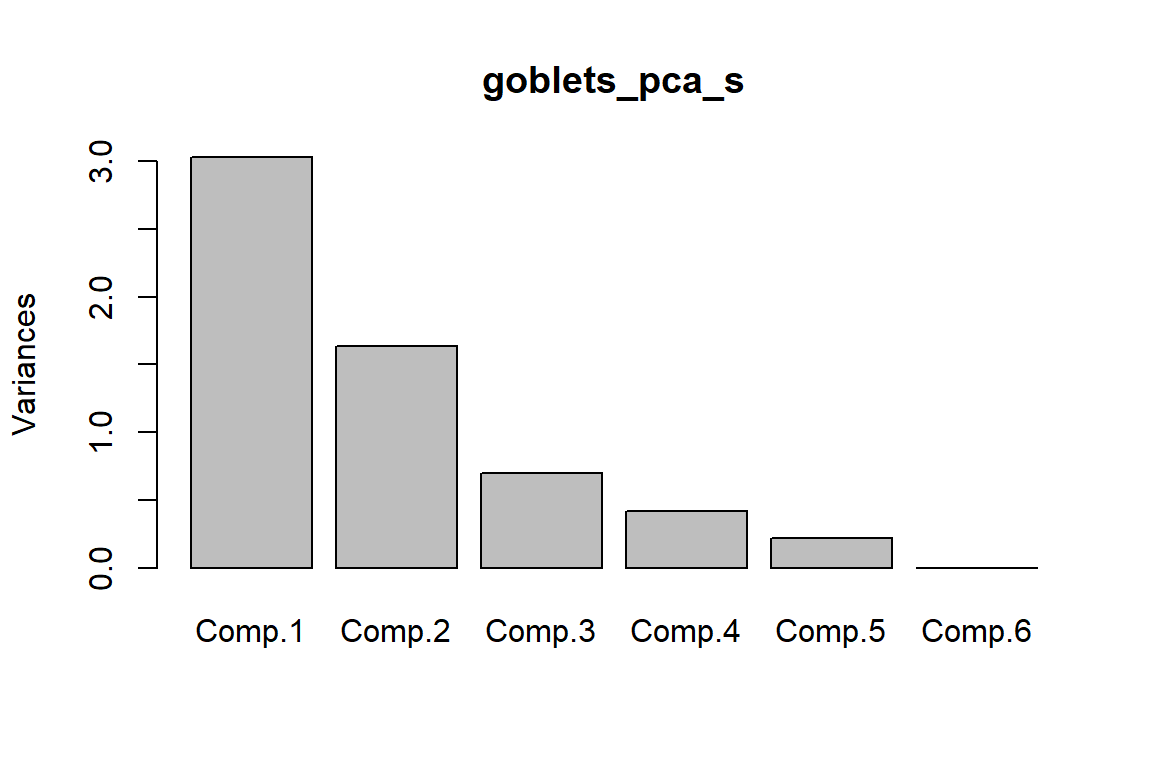How many principal components appear to be sufficient to explain most of the variation in the size-adjusted measurements? Discuss.

## 1.5(2 p) Visualizing PCs

Here are two-dimensional plots of PC1 against PC2, PC1 against PC3, and PC2 against PC3.

library(ggplot2)
p1 <- ggplot(as.data.frame(goblets_pca_s$scores), aes(x = Comp.1, y = Comp.2)) + geom_point() p1 <- p1 + geom_text(aes(label = 1:nrow(goblets_pca_s$scores)), vjust = -0.5, alpha = 0.5)
p2 <- ggplot(as.data.frame(goblets_pca_s$scores), aes(x = Comp.1, y = Comp.3)) + geom_point() p2 <- p2 + geom_text(aes(label = 1:nrow(goblets_pca_s$scores)), vjust = -0.5, alpha = 0.5)
p3 <- ggplot(as.data.frame(goblets_pca_s$scores), aes(x = Comp.2, y = Comp.3)) + geom_point() p3 <- p3 + geom_text(aes(label = 1:nrow(goblets_pca_s$scores)), vjust = -0.5, alpha = 0.5)

library(gridExtra)
grid.arrange(grobs = list(p1, p2, p3), nrow=1, top = "Scatterplots of first three PCs")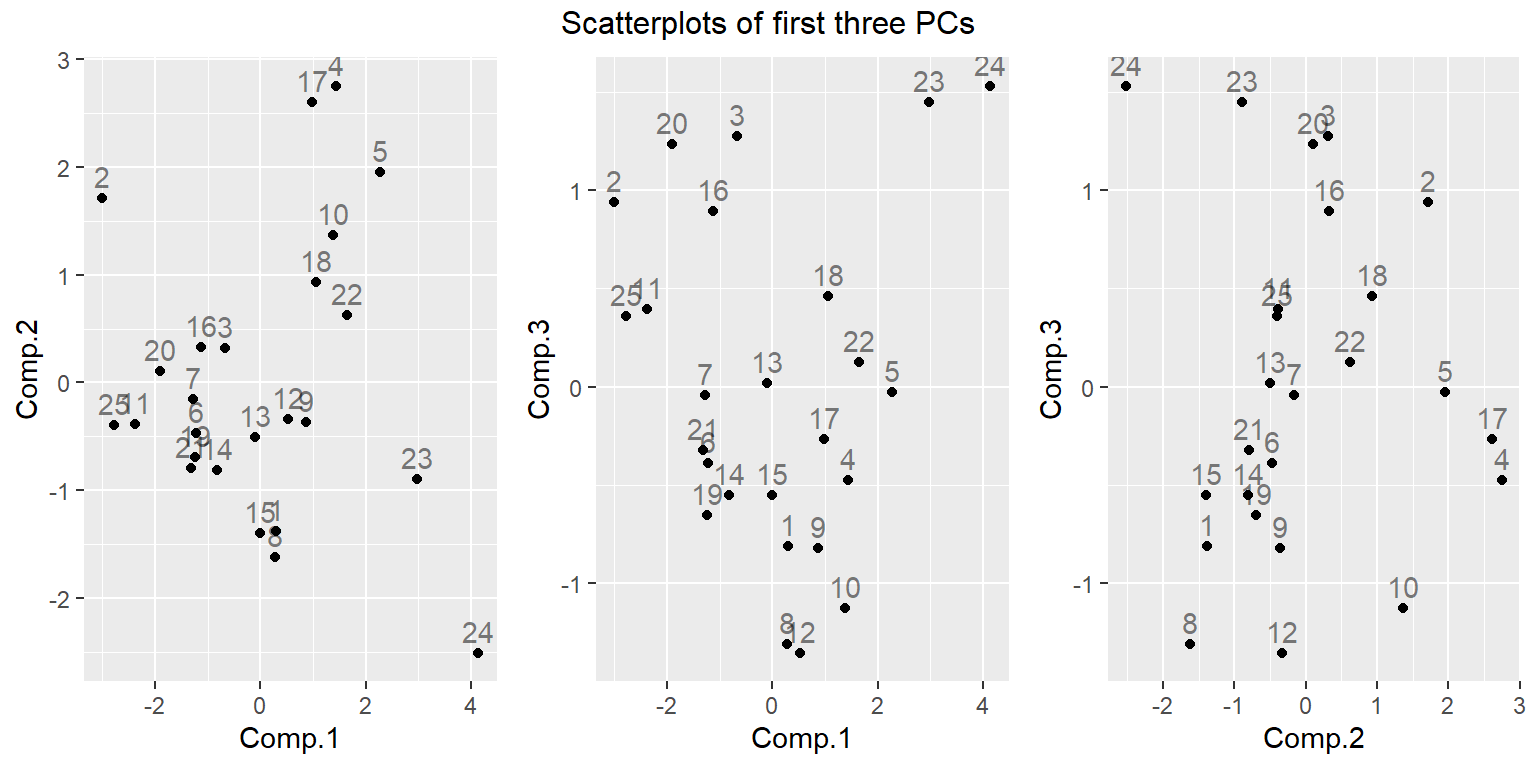Is anything interesting suggested by these plots? Describe how observations cluster or stand out, and what you might wish to know about the goblets because of what you see.